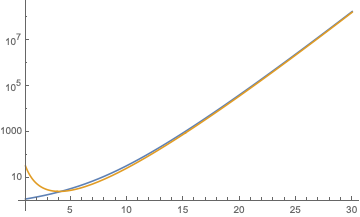# Asymptotic expansion of hypergeometric 2F2

I would like to find an asymptotic expansion for the hypergeometric function

$$_{2}F_{2}\left(a,b;c,d;z\right),\quad a,b,c,d\in\mathbb{R}.$$

The parameters are fixed. $$z$$ is real and $$z\rightarrow +\infty$$.

Could someone shed light on it?

## 1 Answer

$$_{2}F_{2}\left(a,b;c,d;z\right)=\frac{\Gamma (c) \Gamma (d)}{\Gamma (a) \Gamma (b)}e^z z^{a+b-c-d}\left(1+{\cal O}(z^{-1})\right)$$

As an example, the plot shows $$_{2}F_{2}\left(a,b;c,d;z\right)$$ (blue) and the asymptotics (gold) for $$a=1,b=2,c=3,d=4$$.